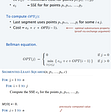# Finding The First Duplicate Value in Python

Here’s a problem that took me a while to figure out an optimal solution.

The First Duplicate Value.

So far, I’ve noticed some techniques that can be applied to data structure and algorithm problems.

For example, the pointer technique, to compare different elements in an array, and creating a hashmap or dictionary (in python), to store checked values.

This problem however, I had to think more creatively and found a way to get an optimized solution that wouldn’t use up more space.

Let’s get started.

# The Prompt

Given an array of integers between 1 and n, inclusive, where n is the length of the array.

Create a function that returns the first integer that appears more than once (left-to-right).

# The Code

We can start by traversing through the input array and looking at each element.

Now we’re looking at each element (value).

The technique we’ll use here goes by the following:

• we’ll check an element.
• Since the array is inclusive, we can go to the element in the array in the index of the absolute value of the current element, minus one.
• Change that element to a negative.
• Now, we’ll traverse through the array. When we come across the negative number, we’ll know that we already checked the same value before. Meaning that first negative instance, will be our first duplicate value.

So, from here we can check if the element at the position of (absolute value of current element) minus (1) is a negative.

If it’s a negative, then that value is the first duplicate. So we can return the absolute value of that element. (Since the first negative will be our answer, we’ll need to get the absolute value and return that).

Now, if the element hasn’t been checked yet. We’ll have to go through the motions and create a negative instance. So, if it’s the first time checking the element, we’ll go to the index of the element in the position of (the absolute value of the current element minus 1), and multiply it by (-1). The next piece of code will look like the following:

Now after this code, if the duplicate hasn’t been found. That means there is no duplicate value, and as per the problem, we need to return a -1. The final code should look like this:

# Complexity Analysis

The time complexity of this solution is O(n) time, where (n) is the length of the input array.

The space complexity of this solution is O(1), constant, space, since we aren’t utilizing any external data structures.

--

--

--

## More from Karan S. Chauhan

Love podcasts or audiobooks? Learn on the go with our new app.

## Flutter — Split View Widget## Setup Local Integration Environment with K3s and Docker Compose## PowerAI the power painter## How to convert a string column to title case, lower case, or upper case with Python, Pandas, and…## The Benefits of a Hybrid Transactional and Analytical Processing Database## HTML Refresher## Use Of Keywords And Identifiers## How to Create Faster Arrays in Python Using NumPy## Snakes and things: Some topics about python and data structure## Implementing a few algorithms with python